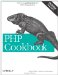# Recipe 2.3. Rounding Floating-Point Numbers

#### 2.3.1. Problem

You want to round a floating-point number, either to an integer value or to a set number of decimal places.

#### 2.3.2. Solution

To round a number to the closest integer, use round( ) :

`\$number = round(2.4);   // \$number = 2`

To round up, use ceil( ) :

`\$number = ceil(2.4);    // \$number = 3`

To round down, use floor( ) :

`\$number = floor(2.4);   // \$number = 2`

#### 2.3.3. Discussion

If a number falls exactly between two integers, PHP rounds away from 0:

`\$number = round(2.5);   //  3 \$number = round(-2.5);  // -3`

You may remember from Recipe 2.2 that floating-point numbers don't always work out to exact values because of how the computer stores them. This can create confusion. A value you expect to have a decimal part of "0.5" might instead be ".499999...9" (with a whole bunch of 9s) or ".500000...1" (with many 0s and a trailing 1).

PHP automatically incorporates a little "fuzz factor " into its rounding calculations, so you don't need to worry about this.

To keep a set number of digits after the decimal point, round( ) accepts an optional precision argument. For example, perhaps you are calculating the total price for the items in a user's shopping cart:

`<?php \$cart = 54.23; \$tax = \$cart * .05; \$total = \$cart + \$tax;       // \$total = 56.9415 \$final = round(\$total, 2);   // \$final = 56.94 ?>`

To round a number down, use the floor( ) function:

`\$number = floor( 2.1);  //  2 \$number = floor( 2.9);  //  2 \$number = floor(-2.1);  // -3 \$number = floor(-2.9);  // -3`

While to round up, use the ceil( ) function:

`\$number = ceil( 2.1);  //  3 \$number = ceil( 2.9);  //  3 \$number = ceil(-2.1);  // -2 \$number = ceil(-2.9);  // -2`

These two functions are named because when you're rounding down, you're rounding "toward the floor," and when you're rounding up, you're rounding "toward the ceiling."

Recipe 2.2 for information on comparing floating-point numbers; documentation on ceil( ) at http://www.php.net/ceil, on floor( ) at http://www.php.net/floor, and on round( ) at http://www.php.net/round .PHP Cookbook: Solutions and Examples for PHP Programmers
ISBN: 0596101015
EAN: 2147483647
Year: 2006
Pages: 445

Similar book on Amazon